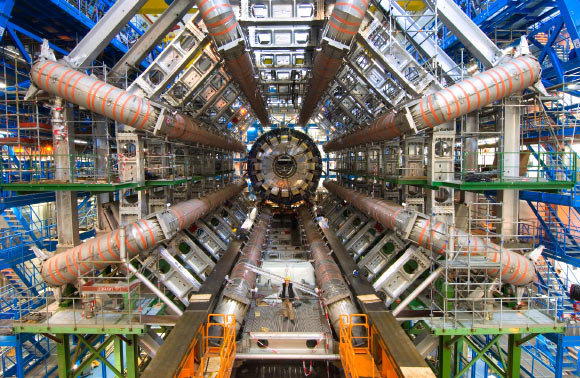# CERN Physicists Test ‘Lepton Flavor Universality’

Physicists from the ATLAS Collaboration at CERN’s Large Hadron Collider (LHC) have successfully performed measurements of ‘lepton flavor universality,’ a fundamental principle of the Standard Model of particle physics. Their results are the first such measurement from LHC, and the most precise one to date, surpassing the precision obtained from experiments at LHC’s predecessor — the Large Electron-Positron Collider.The ATLAS physicists tested the attractive force between muons or τ leptons and another type of fundamental particle, the W boson. Image credit: CERN.

Our understanding of elementary particles and the electromagnetic, weak and strong fundamental forces that act between them is formulated in the Standard Model of particle physics.

Leptons are a type of elementary particle. Electrons, muons and τ leptons represent three varieties (or flavors) of charged leptons.

The Standard Model assumes that the couplings of particles that mediate the weak force — known as ‘W’ or ‘Z’ electroweak gauge bosons — to leptons occurs irrespective of their flavor. This is known as lepton flavor universality.

Physicists from the ATLAS Collaboration studied whether this ‘universal truth’ holds true for the coupling of the muon and the τ lepton to the W boson.

“It is a fundamental axiom of the Standard Model that the couplings of the electroweak gauge bosons (W, Z) to charged leptons, gℓ, are independent of the mass of the leptons,” they explained.

“This fundamental assumption is referred to as ‘lepton-flavor universality’ and is tested in this study by comparing the relative decay rates of on-shell W bosons to τ leptons and muons.”

The researchers obtained a clean sample of W bosons from the decay of top quarks produced in proton-proton collisions and recorded with the ATLAS detector at LHC.

By measuring the ratio of the W decay rates to a τ lepton and to a muon, they were able to conclude that the weak force interacts with both types of lepton in the same way.

“Using 139 fb−1 of proton-proton collisions recorded with the ATLAS detector at a center-of-mass energy of 13 TeV, we report a measurement of this quantity from di-leptonic tt¯ events where the top quarks decay into a W boson and a bottom quark,” they said.

“We can distinguish muons originating from W bosons and those originating from an intermediate τ lepton through the muon transverse impact parameter and differences in the muon transverse momentum spectra.”

“The measured value of R(τ/μ) is 0.992 and is in agreement with the hypothesis of universal lepton couplings as postulated in the Standard Model.”

The results were published in the journal Nature Physics.

_____

G. Aad et al. (ATLAS Collaboration). Test of the universality of τ and μ lepton couplings in W-boson decays with the ATLAS detector. Nat. Phys, published online July 5, 2021; doi: 10.1038/s41567-021-01236-w